﻿ 基于ArcGIS的可拓住宅建筑设计数据库构建研究
«上一篇文章快速检索 高级检索

 智能系统学报2018, Vol. 13Issue (2): 214-219  DOI: 10.11992/tis.2017080060

### 引用本文GAO Zhihui, ZOU Guangtian. Construction of extension residential architectural design database based on ArcGIS[J]. CAAI Transactions on Intelligent Systems, 2018, 13(2): 214-219. DOI: 10.11992/tis.201708006.### 文章历史

1. 哈尔滨工业大学 建筑学院，黑龙江 哈尔滨 150006;
2. 吉林建筑大学城建学院 建筑系，吉林 长春 130021;
3. 哈尔滨工业大学 建筑计划与设计研究所，黑龙江 哈尔滨 150006;
4. 黑龙江省寒地建筑科学重点实验室，黑龙江 哈尔滨 150006

Construction of extension residential architectural design database based on ArcGIS
GAO Zhihui1,2,3,4, ZOU Guangtian1,3,41. School of Architecture, Harbin Institute of Technology, Harbin 150006, China;
2. Department of Architecture, Urban Construction College, Jilin Jianzhu University, Changchun 130021, China;
3. Architectural Planning and Design Institute, Harbin Institute of Technology, Harbin 150006, China;
4. Heilongjiang Cold Region Architecture Science Key Laboratory, Harbin 150006, China
Abstract: This paper proposes a method of constructing the building design database of an extendable house based on the ArcGIS software, and considers the classification expression method, collation of data information, design of database logical structure, and matching method of image and attribute data as the building design data of an extendable residence. A database was constructed on the basis of a residential area instance. The experimental results revealed that the method could convert the residential architectural design image and attribute information into a quantizable and matchable database with a unified format that facilitates computer recognition and could form an efficient house data storage platform. Thus, a rich data basis for the building design of an extendable house and the subsequent process of data mining were provided.
Key words: ArcGIS    residential building design    residential building design data    data integration    extension residential building design    database    extension residential architectural design database    construction

1 可拓住宅建筑设计数据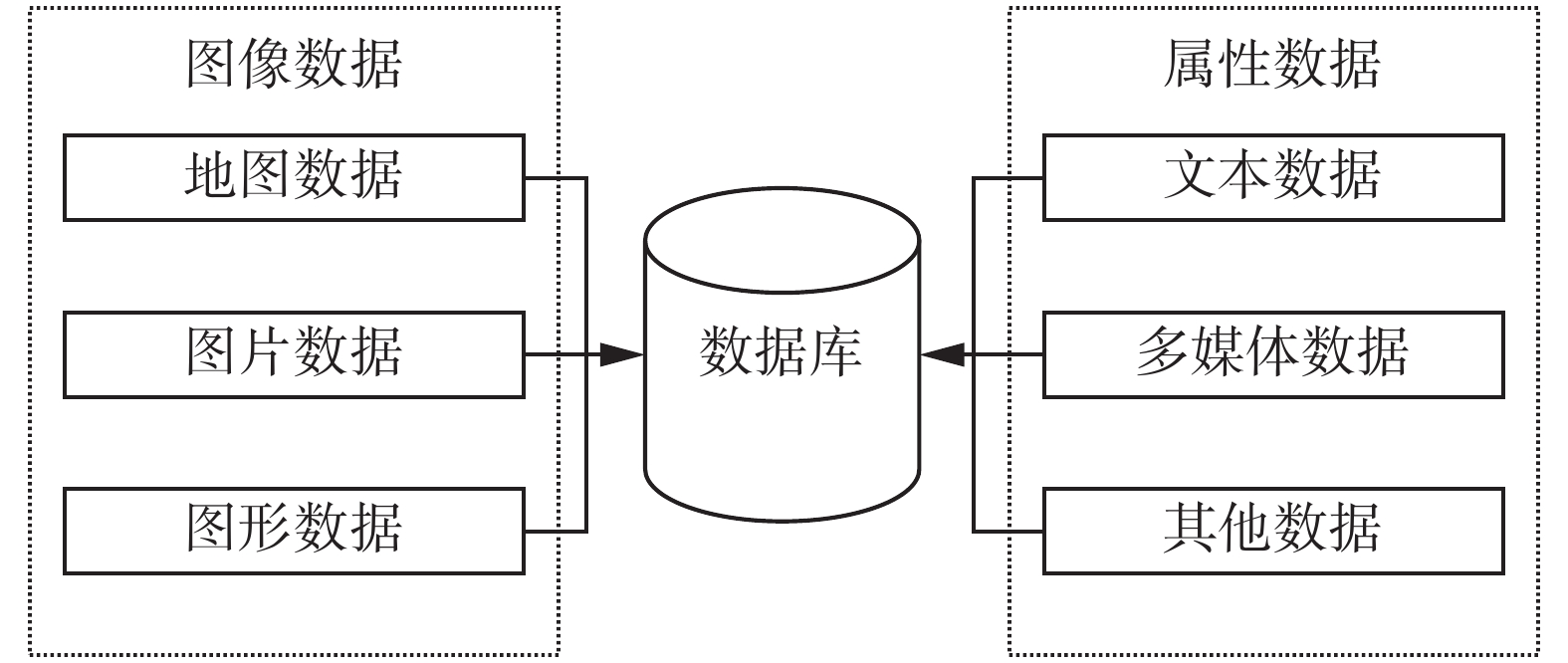Download: 图 1 可拓住宅建筑设计数据组成 Fig. 1 Data composition of extension residential architectural design
1.1 图像数据表达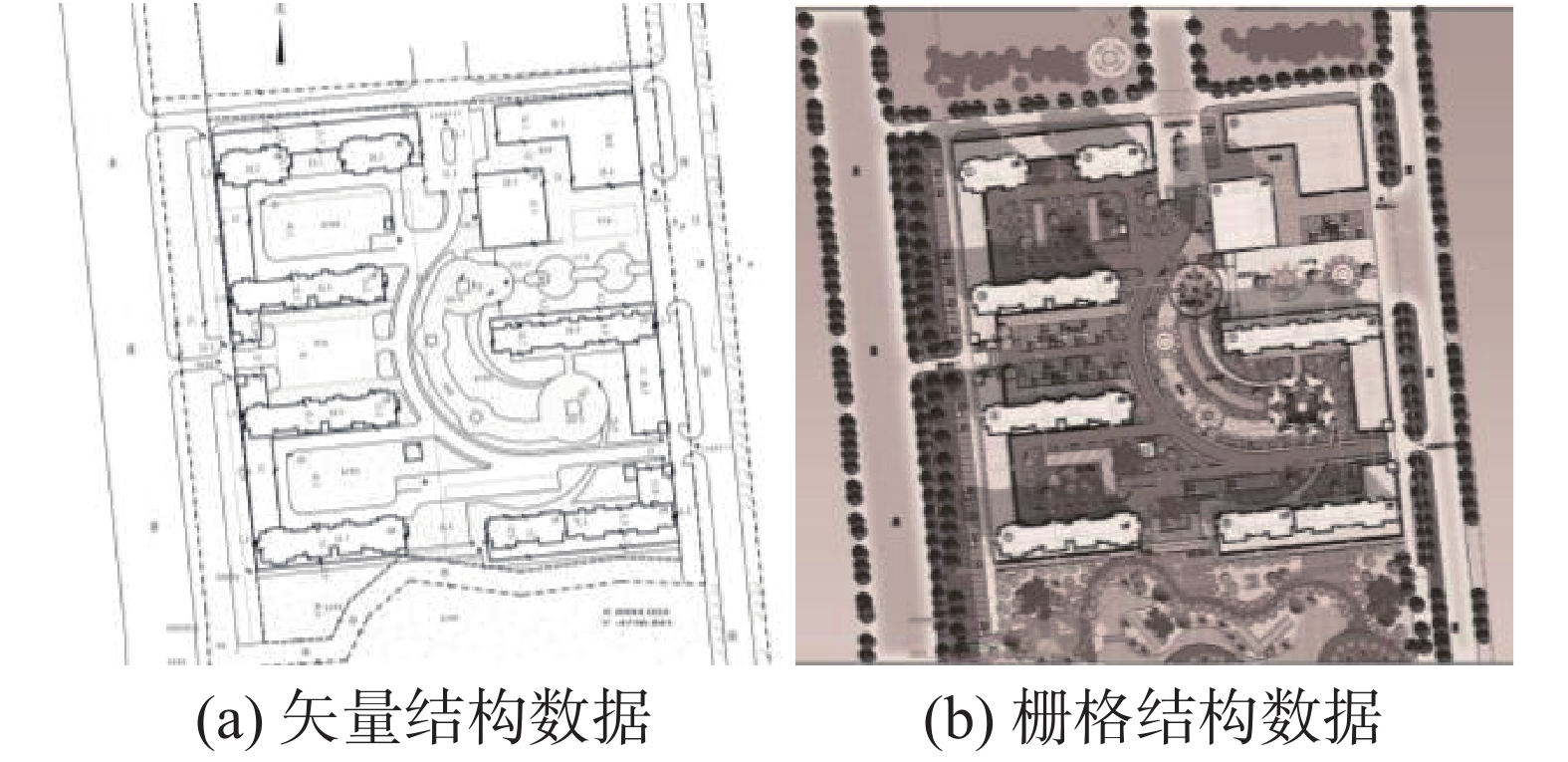Download: 图 2 图像数据类型 Fig. 2 Graphic data type
1.2 属性数据建模

1.2.1 物元表达住宅建筑的基本信息

Om为对象，cm为特征，Om关于cm的量值vm的有序三元组作为描述住宅建筑M的基本信息，用n维物元表示住宅建筑多个特征及其对应的量值，即

 $M = \left( {{O_m},{c_m},{v_m}} \right) = \left[ {\begin{array}{*{20}{c}}{{O_m},}&{{\text{名称}},}&{{v_{m1}}}\\{}&{{\text{位置}},}&{{v_{m2}}}\\{}&{{\text{规模}},}&{{v_{m3}}}\\{\begin{array}{*{20}{c}}{}\\{}\end{array}}&{\begin{array}{*{20}{c}} \vdots \\{{\text{图形}},}\end{array}}&{\begin{array}{*{20}{c}} \vdots \\{{v_{mn}}}\end{array}}\end{array}} \right]$

 ${M_{\text{评价}}} = \left[ {\begin{array}{*{20}{c}}\!\!\!\! {{O_m},}&{\text{评价},}&{\text{很好}} \!\!\!\! \\\!\!\!\! {}&{\text{评价},}&{\text{好}} \!\!\!\! \\\!\!\!\! {}&{\text{评价},}&{\text{一般}} \!\!\!\! \\{\begin{array}{*{20}{c}}{}\\{}\end{array}}&{\begin{array}{*{20}{c}}\!\! {\text{评价},} \!\! \\\!\! {\text{评价},} \!\! \end{array}}&{\begin{array}{*{20}{c}}\!\! {\text{较差}} \!\! \\\!\! {\text{差}} \!\! \end{array}}\end{array}} \right] = \left[ {\begin{array}{*{20}{c}}\!\!\!\! {{O_m},}&{\text{评价},}&5 \!\!\!\!\!\! \\\!\!\!\! {}&{\text{评价},}&4 \!\!\!\!\!\! \\\!\!\!\! {}&{\text{评价},}&3 \!\!\!\!\!\! \\\!\!\!\! {}&{\text{评价},}&2 \!\!\!\!\!\! \\\!\!\!\! {}&{\text{评价},}&\,\,\,\,1 \end{array}} \right]$
1.2.2 关系元表达住宅建筑的关系信息

 $R = \left( {o,c,v} \right) = \left[ {\begin{array}{*{20}{c}}{\text{位置关系},}&{\text{前项},}&\text{别墅}\\{}&{\text{后项},}&\text{溪流}\\{}&{\text{方位},}&\text{上方}\\{}& \vdots & \vdots\end{array}} \right]$

1.3 数据信息整理

1.3.1 图像数据整理

ArcGIS提供了空间拓扑分析功能，有去除冗余顶点、重复线、节点类型识别等；图面上有水平或垂直的直线条，以这根直线做参考进行矫正；对于地图的横、纵向误差，通过扫描图长宽尺度与地图实际尺寸相比较进行纠正；对于遥感影像在进行解译时，一般选用和遥感影像比例尺相近的地形图或正投影，做重合比对分别纠正。

1.3.2 属性数据整理

2 可拓住宅建筑设计数据库

1)用可拓学语言描述建筑设计相关要素，整合数据并构建统一的表达语言，便于计算机识别；

2)形成高效的住宅数据存储平台，为可拓住宅建筑设计和后续的数据挖掘过程提供丰富的数据基础。

3 可拓住宅建筑设计数据库构建方法 3.1 逻辑结构设计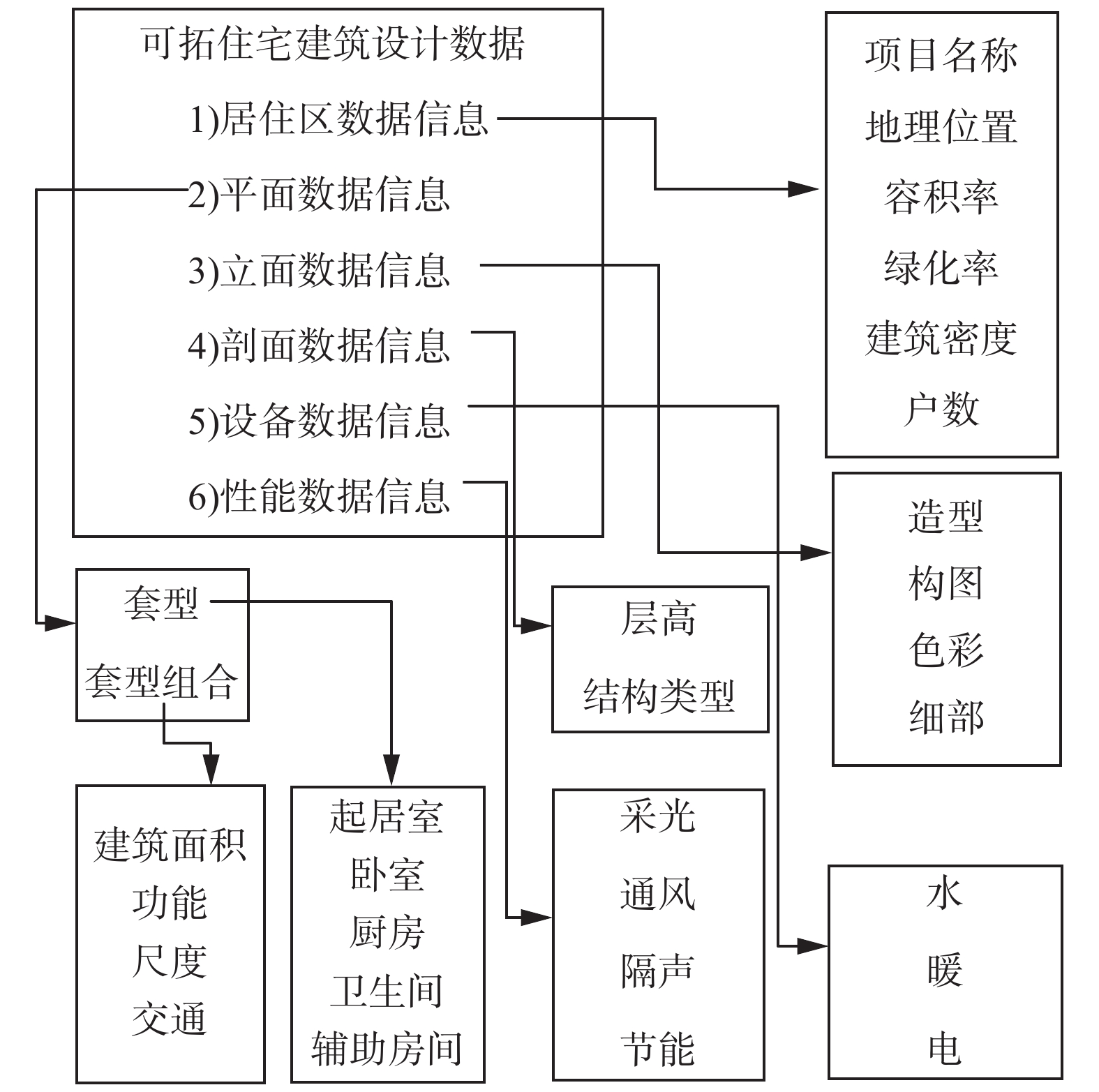Download: 图 3 可拓住宅建筑设计数据建模 Fig. 3 Model building of extension residential architectural design database

3.2 图像数据与属性数据的匹配

4 实验与分析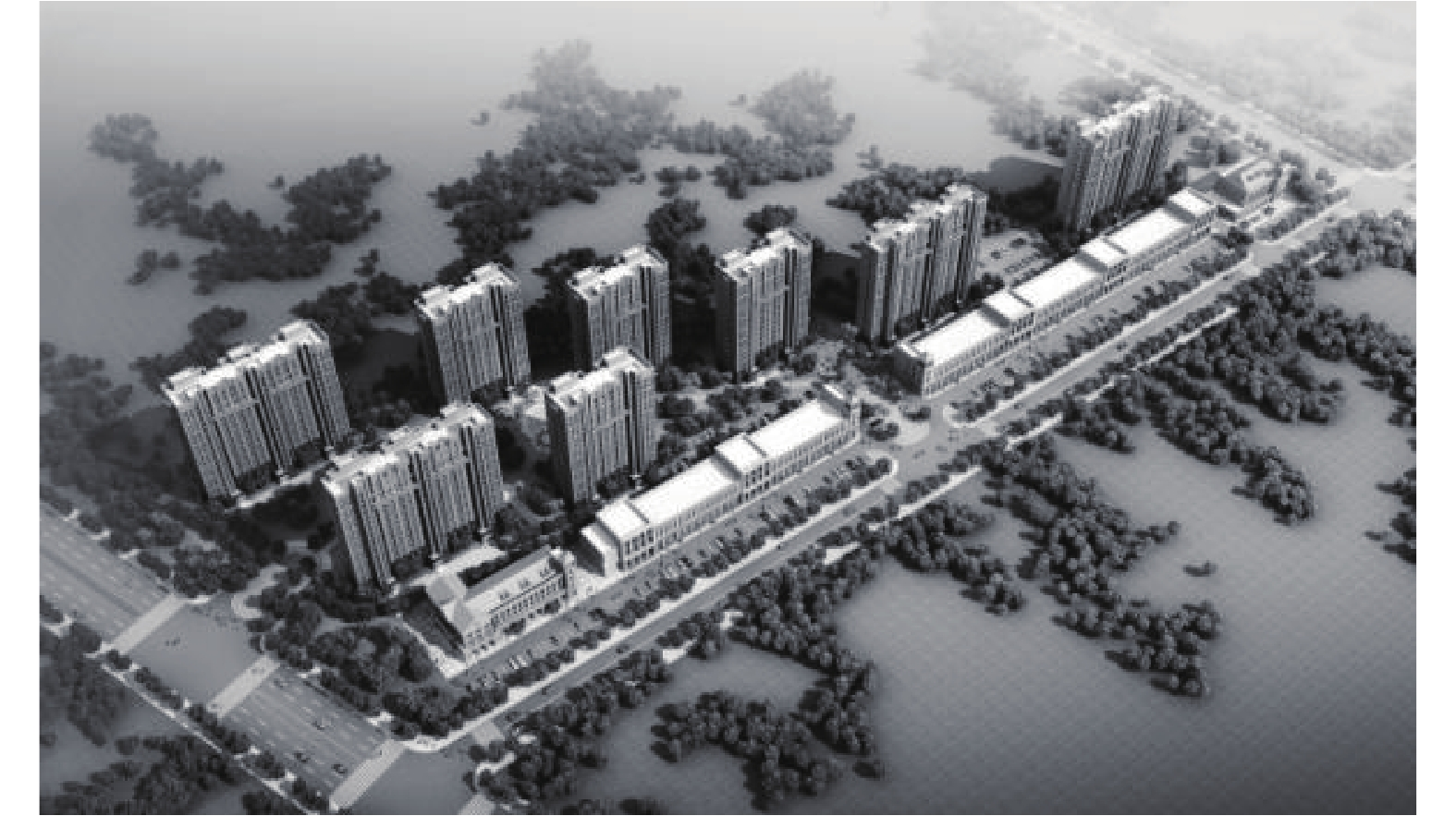Download: 图 4 某居住小区项目 Fig. 4 The project of a residential district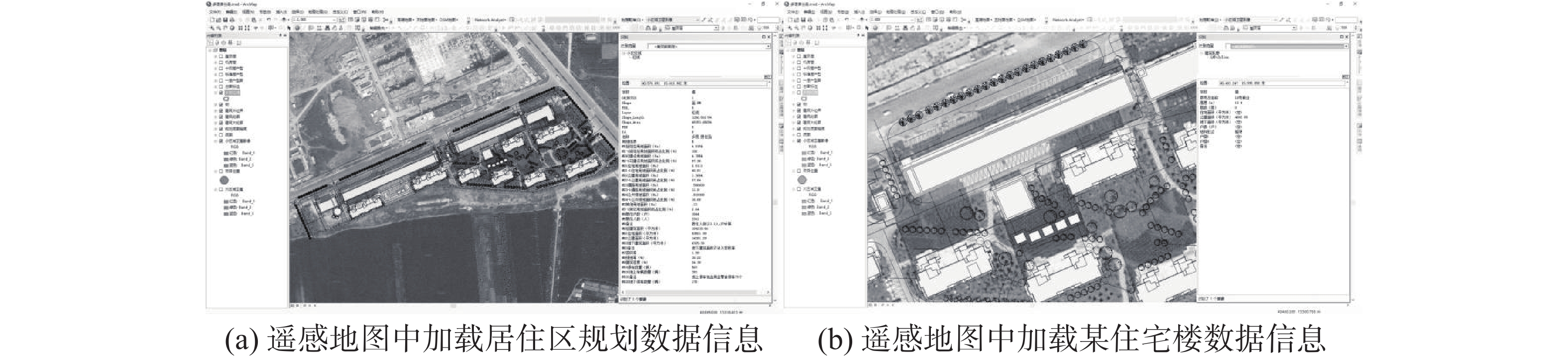Download: 图 5 ArcGIS软件中加载居住区住宅建筑数据信息 Fig. 5 ArcGIS loaded residential area planning data information

 ${M_{\text{居住区}}} = \left[ {\begin{array}{*{20}{l}}\text{居住区},&\text{项目名称},&\text{某居住小区}\\{}&\text{项目类型}&\text{普通商住小区}\\{}&{\text{建设用地},}&{4.785\;4}\\{}&{\text{容积率},}&{1.99}\\{}&{\text{绿化率},}&{30.22\% }\\{}&{\text{建筑密度},}&{24.39\% }\\{}&{\vdots}&{\vdots}\end{array}} \right]$
 ${M_{1\text{号楼}}} = \left[ {\begin{array}{*{20}{l}}{\begin{array}{*{20}{c}}{\begin{array}{*{20}{c}}\!\!\!\!\!\!\!\!{{O_M},}\\{}\end{array}}\\{}\end{array}}&{\begin{array}{*{20}{c}}{\begin{array}{*{20}{c}}{\text{高度},}\\{\text{层数},}\end{array}}\\{\text{面积},}\end{array}}&{\begin{array}{*{20}{l}}{\begin{array}{*{20}{l}}{50.95}\\{18}\end{array}}\\{12\;591.06}\end{array}}\\{}&{\text{套型组合方式},}&{{M_{11}} \cap {M_{12}} \cap {M_{11}}}\\{}&{\text{户数},}&{162}\\{}&{\text{结构形式},}&\text{剪力墙}\end{array}} \right]$

 ${M_{11}} = \left[ {\begin{array}{*{20}{l}}{{O_M},}&{\text{户型},}&{2 - 2 - 1}\\{}&{\text{建筑面积},}&{91.32}\\{}&{\text{使用面积},}&{71.40}\end{array}} \right]$
 ${M_{12}} = \left[ {\begin{array}{*{20}{l}}{{O_M},}&{\text{户型},}&{1 - 1 - 1}\\{}&{\text{建筑面积},}3&{49.65}\\{}&{\text{使用面积},}&{39.14}\end{array}} \right]$
5 结论

  杨春燕, 李小妹, 陈文伟, 等. 可拓数据挖掘方法及其计算机实现[M]. 广东: 广东高等教育出版社, 2010: 25–32. YANG Chunyan, LI Xiaomei, CHEN Wenwei, et al. Extension data mining method and its computer implementation[M]. Guangdong: Guangdong higher education press, 2010: 25–32. (0)  陈文伟, 黄金才. 可拓集与可拓数据挖掘[M]. 北京: 科学出版社, 2008: 37–41. CHEN Wenwei, HUANG Jincai. Extension set and extension data mining[M]. Beijing: Science press, 2008: 37–41. (0)  蔡文, 杨春燕, 何斌. 可拓逻辑初步[M]. 北京: 科学出版社, 2003: 15–17. CAI Wen, YANG Chunyan, HE Bin. The preliminary extension logic[M]. Beijing: Science press, 2003: 15–17. (0)  王珊, 萨师煊, 张俊. 数据库系统概论[M]. 北京: 高等教育出版社, 2014. WANG Sa, SA Shixuan, ZHANG Jun. Introduction of database system[M]. Beijing: Higher education press, 2014. (0)  曾维, 魏蛟龙. 可拓知识库的构建[J]. 船舶电子工程, 2004, 3: 53-55. ZENG Wei, WEI Jiaolong. Extension repository designing[J]. Ship electronic engineering, 2004, 3: 53-55. (0)  豆增发, 王保保. 基于可拓学的数据库建模方法[J]. 西安邮电学院学报, 2006, 9: 79-82. DOU Zengfa, WANG Baobao. The method of database modelling based on extension theory[J]. Journal of Xi’an university of post and telecommunications, 2006, 9: 79-82. (0)  张铭, 曹鸿飞. 城市轨道交通线网数据中心与评估决策平台[J]. 智能系统学报, 2017, 12(3): 1-9. ZHANG Ming, CAO Hongfei. The platform of data center and evaluation decision making of urban rail transit[J]. CAAI transactions on intelligent systems, 2017, 12(3): 1-9. (0)  谢颖, 张毅, 操骏峰, 等. 多媒体数据库研究[J]. 重庆邮电大学学报: 自然科学版, 2017, S1: 134-137. XIE Ying, ZHANG Yi, CAO Junfeng, et al. Research of multimedia database[J]. Journal of Chongqing university of posts and telecommunications: natural science, 2017, S1: 134-137. (0)  赵燕伟, 周建强, 洪欢欢. 可拓设计理论方法综述与展望[J]. 计算机集成制造系统, 2015, 5: 1157-1167. ZHAO Yanwei, ZHOU Jianqiang, HONG Huanhuan. Overview and prospects of extension design methodology[J]. Computer integrated manufacturing systems, 2015, 5: 1157-1167. (0)  李江, 周浩, 罗吴亮. ArcGIS空间数据库中拓扑错误的检查与批量处理方法[J]. 北京测绘, 2017, 2: 156-157. LI Jiang, ZHOU Hao, LUO Wuliang. Research on the conversion of DLG data to data factors of important geographical conditions[J]. Beijing surveying and mapping, 2017, 2: 156-157. (0)  李潇潇. 简述ArcGIS在DEM数据格式转换及DEM、DOM数据质量检查过程中的应用[J]. 测绘与空间地理信息, 2015, 2: 5-8. LI Xiaoxiao. Described on the application of ArcGIS in DEM data format conversion, DEM and DOM data quality inspection process[J]. Geomatics and spatial information technology, 2015, 2: 5-8. DOI:10.3969/j.issn.1672-5867.2015.02.002 (0)  孙霞, 曹刚, 王伟东. ArcGIS影像管理解决方案浅析[J]. 测绘与空间地理信息, 2017, 1: 175-176. SUN Xia, CAO Gang, WANG Weidong. Analysis of ArcGIS image management solution[J]. Geomatics and spatial information technology, 2017, 1: 175-176. (0)  段正励, 刘抚英. 杭州市工业遗产综合信息数据库构建研究[J]. 建筑学报, 2013, 10: 47. DUAN Zhengli, LIU Fuying. Study on the comprehensive information database of industrial heritage in hangzhou[J]. Architectural Journal, 2013, 10: 47. (0)